Pearson correlation vs simple linear regressionDr. Vanessa Cave

7 months ago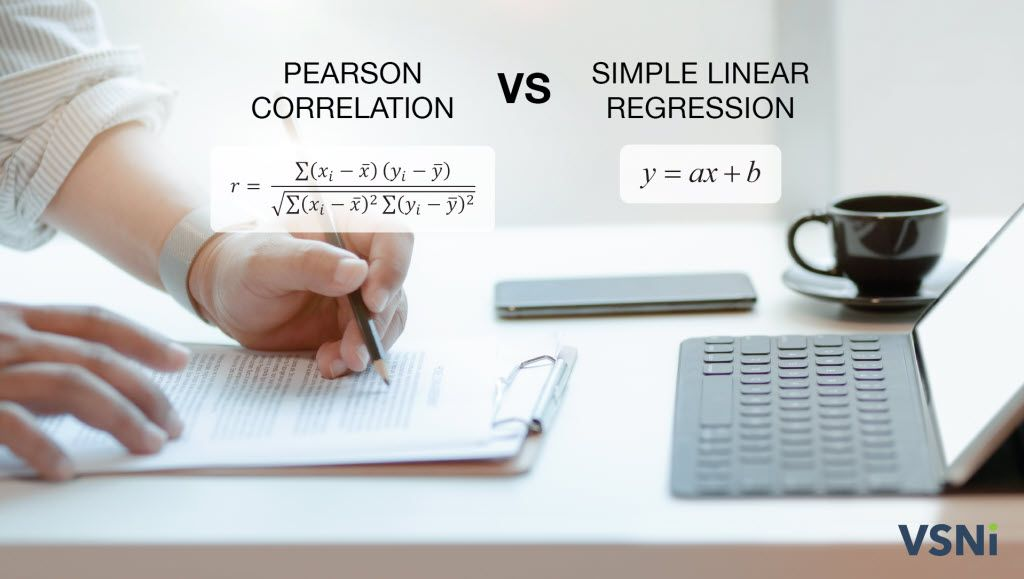Both Pearson correlation and simple linear regression can be used to determine if two numeric variables are linearly related. Nevertheless, there are important differences between these two approaches.

• Pearson correlation is a measure of the strength and direction of the linear association between two numeric variables that makes no assumption of causality.
• Simple linear regression describes the linear relationship between a response variable (denoted by ) and an explanatory variable (denoted by ) using a statistical model, and this model can be used to make predictions.

The following table summarizes the key similarities and differences between Pearson correlation and simple linear regression.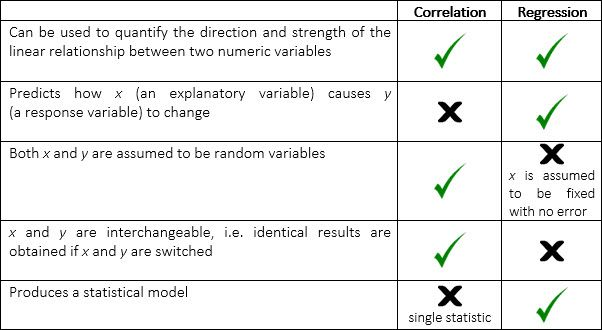Pearson correlation

Pearson correlation is a number ranging from -1 to 1 that represents the strength of the linear relationship between two numeric variables. A value of 1 corresponds to a perfect positive linear relationship, a value of 0 to no linear relationship, and a value of -1 to a perfect negative relationship.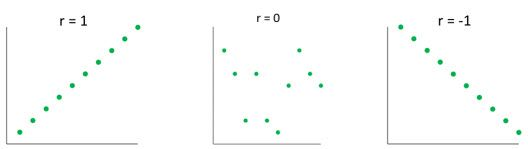The Pearson correlation between variables and is calculated using the formula:

r=fracsumi=1n (xi-barx)(yi-bary)sqrtsumi=1n (xi-barx)2sumi=1n(y_i-bary)2
r = \frac{\sum_{i=1}^n (x_i-\bar x)(y_i-\bar y)}{\sqrt{\sum_{i=1}^n (x_i-\bar x)^2\sum_{i=1}^n(y_i-\bar y)^2}}

where  is the mean of the values, and  is the mean of the values, and is the sample size.

The Pearson correlation is also known as the product-moment correlation coefficient or simply the correlation.

Simple linear regression

If we are interested in the effect of an variate (i.e. a numeric explanatory or independent variable) on a variate (i.e. a numeric response or dependent variable) regression analysis is appropriate. Simple linear regression describes the response variable by the model:

y=a+bx
y = a + bx

where the coefficients and   are the intercept and slope of the regression line respectively. The intercept is the value of when is zero. The slope is the change in for every one unit change in . When the correlation is positive, the slope of the regression line will be positive and vice versa.

The above model is theoretical, and in practice there will be error. The statistical model is given by:

y=hata+hatbx+epsilon
y = \hat a + \hat bx + \epsilon

where , a variate of residuals, represents the difference between the predicted and observed values. The “hat” (^) accent is used to denote values estimated from the observed data. The regression coefficients, and , are estimated by least squares. This results in a regression line that minimizes the sum of squared residuals.

Example using Genstat

 Check out our Genstat tutorial video on Pearson correlation vs simple linear regression.

You can calculate the Pearson correlation, or fit a simple linear regression, using any general statistical software package.  Here, we’re going to use Genstat.

Example: A concrete manufacturer wants to know if the hardness of their concrete depends on the amount of cement used to make it. They collected data from thirty batches of concrete:

 Amount of cement(x) Hardness of concrete(y) Amount of cement (x) Hardness of concrete (y) 16.3 58.1 14.7 46.3 23.2 80.1 20.2 65.9 18.2 47.4 19.5 65.5 20.8 55.5 20.5 53.5 21.9 65.3 25.6 77.7 23.2 72.7 24.4 73.7 17.8 65.5 21.7 64.9 24.2 81.8 18.3 57.5 16.2 53.7 20.0 62.8 23.2 61.1 20.7 58.4 20.4 58.7 24.4 70.5 14.6 52.0 25.8 78.6 17.7 58.8 20.6 62.0 15.9 56.4 17.5 55.5 15.6 49.4 26.3 67.7

The scatterplot of the data suggests that the two variables are linearly related: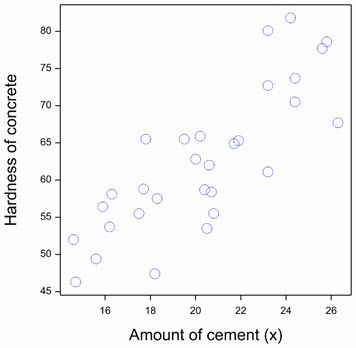Let’s first assess whether there is evidence of a significant Pearson correlation between the hardness of the concrete and the amount of cement used to make it. Our null hypothesis is that true correlation equals zero. Using Genstat, we can see that the correlation estimated from the data is 0.82 with a p-value of <0.001. That is, there is strong statistical evidence of a linear relationship between the two variables.

Here we see the Correlations menu settings and the output this produces in Genstat: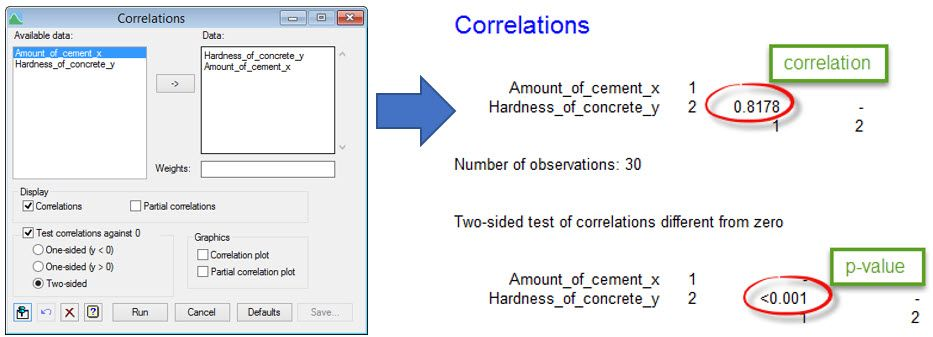Note, the validity of our hypothesis test depends on several assumptions, including that and are continuous, jointly normally distributed (i.e. bivariate Normal), random variables.  If the scatterplot indicates a non-linear relationship between and , the bivariate Normal assumption is violated. You should also check whether both the and variables appear to be normally distributed. This can be done graphically (e.g. by inspecting a boxplot, histogram or Q-Q plot) or with a hypothesis test (e.g. the Shapiro-Wilk test). For both variables in our dataset, neither their boxplot nor the Shapiro-Wilk test indicates a lack of normality.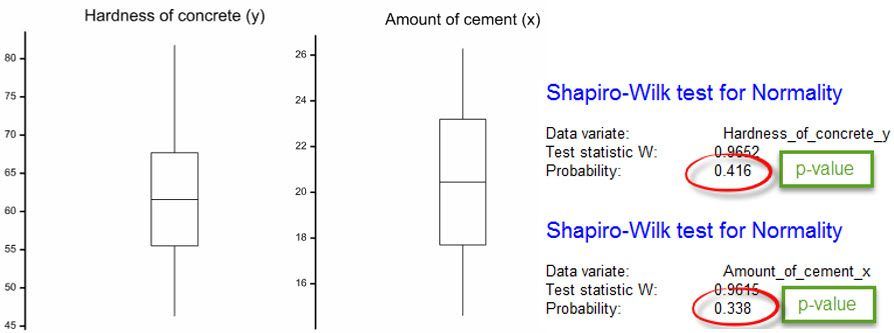As the hardness of the concrete is assumed to represent a response to changes in the amount of cement, it is more informative to model the data using a simple linear regression.

Here we see the Linear Regression and Linear Regression Options menu settings and the output these produce in Genstat: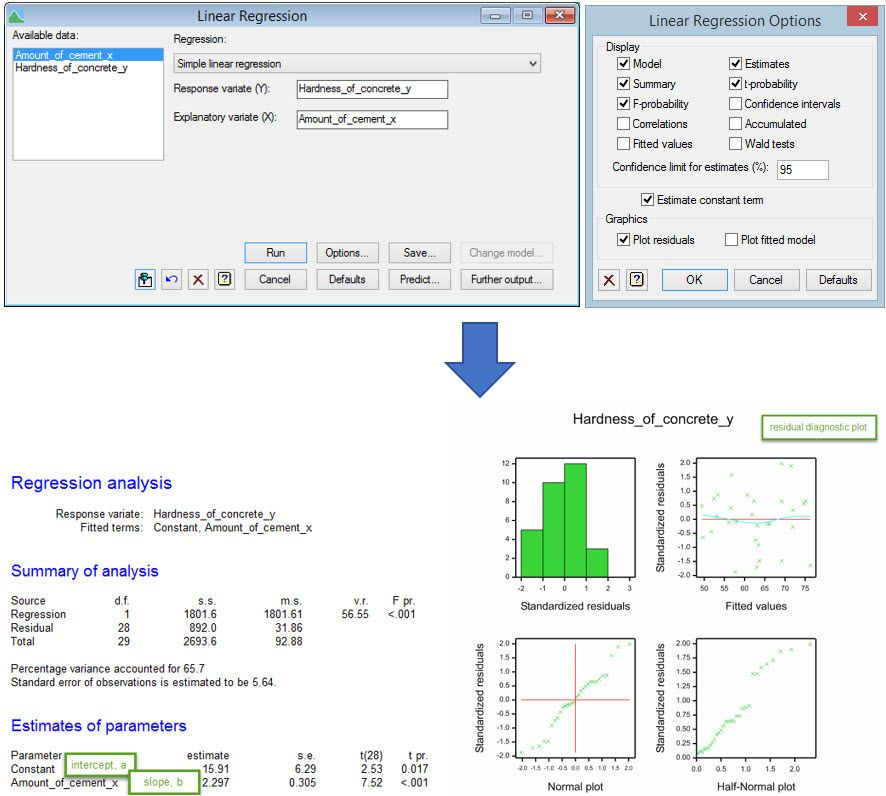Using Genstat, we obtain the following regression line:

Predicted hardness of concrete = 15.91 + 2.297 x amount of cement

That is, the model predicts that for every one unit increase in the amount of cement used, the hardness of the concrete produced increases by 2.297 units. The predicted hardness with 20 units of cement is 15.91 + (2.297 x 20) = 61.85 units.

For a simple linear regression, we are also interested in whether there is evidence of a linear relationship with the explanatory variable. This can be assessed using the variance ratio (v.r.) which, under the null hypothesis of no linear relationship, has an F distribution. The p-value from this test is <0.001, providing strong statistical evidence of a relationship. The percentage variance accounted for summarizes how much variability in the data is explained by the regression model - in this example 65.7%.

The residuals from the regression model are assumed be independent and normally distributed with constant variance. The residual diagnostic plot (see above) is useful for helping check these assumptions. The histogram (top left), Normal plot (bottom left) and half-Normal plot (bottom right) are used to assess normality; the histogram should be reasonably symmetric and bell-shaped, both the Normal plot and half-Normal plot should form roughly a straight line. The scatterplot of residuals against fitted values (top right) is used to assess the constant variance assumption; the spread of the residuals should be equal over the range of fitted values. It can also reveal violations of the independence assumption or a lack of fit; the points should be randomly scattered without any pattern. For our example, the residual diagnostic plot looks adequate.

Dr Vanessa Cave is an applied statistician, interested in the application of statistics to the biosciences, in particular agriculture and ecology. She is a team leader of Data Science at AgResearch Ltd, New Zealand's government-funded agricultural institute, and is a developer of the Genstat statistical software package. Vanessa is currently President of the Australasian Region of the International Biometric Society, past-President of the New Zealand Statistical Association, an Associate Editor for the Agronomy Journal, on the Editorial Board of The New Zealand Veterinary Journal and a member of the Data Science Industry Advisory Group for the University of Auckland. She has a PhD in statistics from the University of St Andrew.

Vanessa has over a decade of experience collaborating with scientists, using statistics to solve real-world problems.  She provides expertise on experiment and survey design, data collection and management, statistical analysis, and the interpretation of statistical findings. Her interests include statistical consultancy, mixed models, multivariate methods, statistical ecology, statistical graphics and data visualisation, and the statistical challenges related to digital agriculture.Dr. John Rogers

10 months ago

50 years of bioscience statistics

Earlier this year I had an enquiry from Carey Langley of VSNi as to why I had not renewed my Genstat licence. The truth was simple – I have decided to fully retire after 50 years as an agricultural entomologist / applied biologist / consultant. This prompted some reflections about the evolution of bioscience data analysis that I have experienced over that half century, a period during which most of my focus was the interaction between insects and their plant hosts; both how insect feeding impacts on plant growth and crop yield, and how plants impact on the development of the insects that feed on them and on their natural enemies.

Where it began – paper and post

My journey into bioscience data analysis started with undergraduate courses in biometry – yes, it was an agriculture faculty, so it was biometry not statistics. We started doing statistical analyses using full keyboard Monroe calculators (for those of you who don’t know what I am talking about, you can find them here).  It was a simpler time and as undergraduates we thought it was hugely funny to divide 1 by 0 until the blue smoke came out…

After leaving university in the early 1970s, I started working for the Agriculture Department of an Australian state government, at a small country research station. Statistical analysis was rudimentary to say the least. If you were motivated, there was always the option of running analyses yourself by hand, given the appearance of the first scientific calculators in the early 1970s. If you wanted a formal statistical analysis of your data, you would mail off a paper copy of the raw data to Biometry Branch… and wait.  Some months later, you would get back your ANOVA, regression, or whatever the biometrician thought appropriate to do, on paper with some indication of what treatments were different from what other treatments.  Dose-mortality data was dealt with by manually plotting data onto probit paper.

Enter the mainframe

In-house ANOVA programs running on central mainframes were a step forward some years later as it at least enabled us to run our own analyses, as long as you wanted to do an ANOVA…. However, it also required a 2 hours’ drive to the nearest card reader, with the actual computer a further 1000 kilometres away.… The first desktop computer I used for statistical analysis was in the early 1980s and was a CP/M machine with two 8-inch floppy discs with, I think, 256k of memory, and booting it required turning a key and pressing the blue button - yes, really! And about the same time, the local agricultural economist drove us crazy extolling the virtues of a program called Lotus 1-2-3!

Having been brought up on a solid diet of the classic texts such as Steele and Torrie, Cochran and Cox and Sokal and Rohlf, the primary frustration during this period was not having ready access to the statistical analyses you knew were appropriate for your data. Typical modes of operating for agricultural scientists in that era were randomised blocks of various degrees of complexity, thus the emphasis on ANOVA in the software that was available in-house. Those of us who also had less-structured ecological data were less well catered for.

My first access to a comprehensive statistics package was during the early to mid-1980s at one of the American Land Grant universities. It was a revelation to be able to run virtually whatever statistical test deemed necessary. Access to non-linear regression was a definite plus, given the non-linear nature of many biological responses. As well, being able to run a series of models to test specific hypotheses opened up new options for more elegant and insightful analyses. Looking back from 2021, such things look very trivial, but compared to where we came from in the 1970s, they were significant steps forward.

Enter Genstat

My first exposure to Genstat, VSNi’s stalwart statistical software package, was Genstat for Windows, Third Edition (1997). Simple things like the availability of residual plots made a difference for us entomologists, given that much of our data had non-normal errors; it took the guesswork out of whether and what transformations to use. The availability of regressions with grouped data also opened some previously closed doors.

After a deviation away from hands-on research, I came back to biological-data analysis in the mid-2000s and found myself working with repeated-measures and survival / mortality data, so ventured into repeated-measures restricted maximum likelihood analyses and generalised linear mixed models for the first time (with assistance from a couple of Roger Payne’s training courses in Hobart and Queenstown). Looking back, it is interesting how quickly I became blasé about such computationally intensive analyses that would run in seconds on my laptop or desktop, forgetting that I was doing ANOVAs by hand 40 years earlier when John Nelder was developing generalised linear models. How the world has changed!

Partnership and support

Of importance to my Genstat experience was the level of support that was available to me as a Genstat licensee. Over the last 15 years or so, as I attempted some of these more complex analyses, my aspirations were somewhat ahead of my abilities, and it was always reassuring to know that Genstat Support was only ever an email away. A couple of examples will flesh this out.

Back in 2008, I was working on the relationship between insect-pest density and crop yield using R2LINES, but had extra linear X’s related to plant vigour in addition to the measure of pest infestation. A support-enquiry email produced an overnight response from Roger Payne that basically said, “Try this”. While I slept, Roger had written an extension to R2LINES to incorporate extra linear X’s. This was later incorporated into the regular releases of Genstat. This work led to the clearer specification of the pest densities that warranted chemical control in soybeans and dry beans (https://doi.org/10.1016/j.cropro.2009.08.016 and https://doi.org/10.1016/j.cropro.2009.08.015).

More recently, I was attempting to disentangle the effects on caterpillar mortality of the two Cry insecticidal proteins in transgenic cotton and, while I got close, I would not have got the analysis to run properly without Roger’s support. The data was scant in the bottom half of the overall dose-response curves for both Cry proteins, but it was possible to fit asymptotic exponentials that modelled the upper half of each curve. The final double-exponential response surface I fitted with Roger’s assistance showed clearly that the dose-mortality response was stronger for one of the Cry proteins than the other, and that there was no synergistic action between the two proteins (https://doi.org/10.1016/j.cropro.2015.10.013

The value of a comprehensive statistics package

One thing that I especially appreciate about having access to a comprehensive statistics package such as Genstat is having the capacity to tease apart biological data to get at the underlying relationships. About 10 years ago, I was asked to look at some data on the impact of cold stress on the expression of the Cry2Ab insecticidal protein in transgenic cotton. The data set was seemingly simple - two years of pot-trial data where groups of pots were either left out overnight or protected from low overnight temperatures by being moved into a glasshouse, plus temperature data and Cry2Ab protein levels. A REML analysis, and some correlations and regressions enabled me to show that cold overnight temperatures did reduce Cry2Ab protein levels, that the effects occurred for up to 6 days after the cold period and that the threshold for these effects was approximately 14 Cº (https://doi.org/10.1603/EC09369). What I took from this piece of work is how powerful a comprehensive statistics package can be in teasing apart important biological insights from what was seemingly very simple data. Note that I did not use any statistics that were cutting edge, just a combination of REML, correlation and regression analyses, but used these techniques to guide the dissection of the relationships in the data to end up with an elegant and insightful outcome.

Final reflections

Looking back over 50 years of work, one thing stands out for me: the huge advances that have occurred in the statistical analysis of biological data has allowed much more insightful statistical analyses that has, in turn, allowed biological scientists to more elegantly pull apart the interactions between insects and their plant hosts.

For me, Genstat has played a pivotal role in that process. I shall miss it.

Dr John Rogers

Research Connections and Consulting

St Lucia, Queensland 4067, Australia

Phone/Fax: +61 (0)7 3720 9065

Mobile: 0409 200 701

Email: john.rogers@rcac.net.au

Alternate email: D.John.Rogers@gmail.comKanchana Punyawaew

a year ago

Linear mixed models: a balanced lattice square

This blog illustrates how to analyze data from a field experiment with a balanced lattice square design using linear mixed models. We’ll consider two models: the balanced lattice square model and a spatial model.

The example data are from a field experiment conducted at Slate Hall Farm, UK, in 1976 (Gilmour et al., 1995). The experiment was set up to compare the performance of 25 varieties of barley and was designed as a balanced lattice square with six replicates laid out in a 10 x 15 rectangular grid. Each replicate contained exactly one plot for every variety. The variety grown in each plot, and the coding of the replicates and lattice blocks, is shown in the field layout below: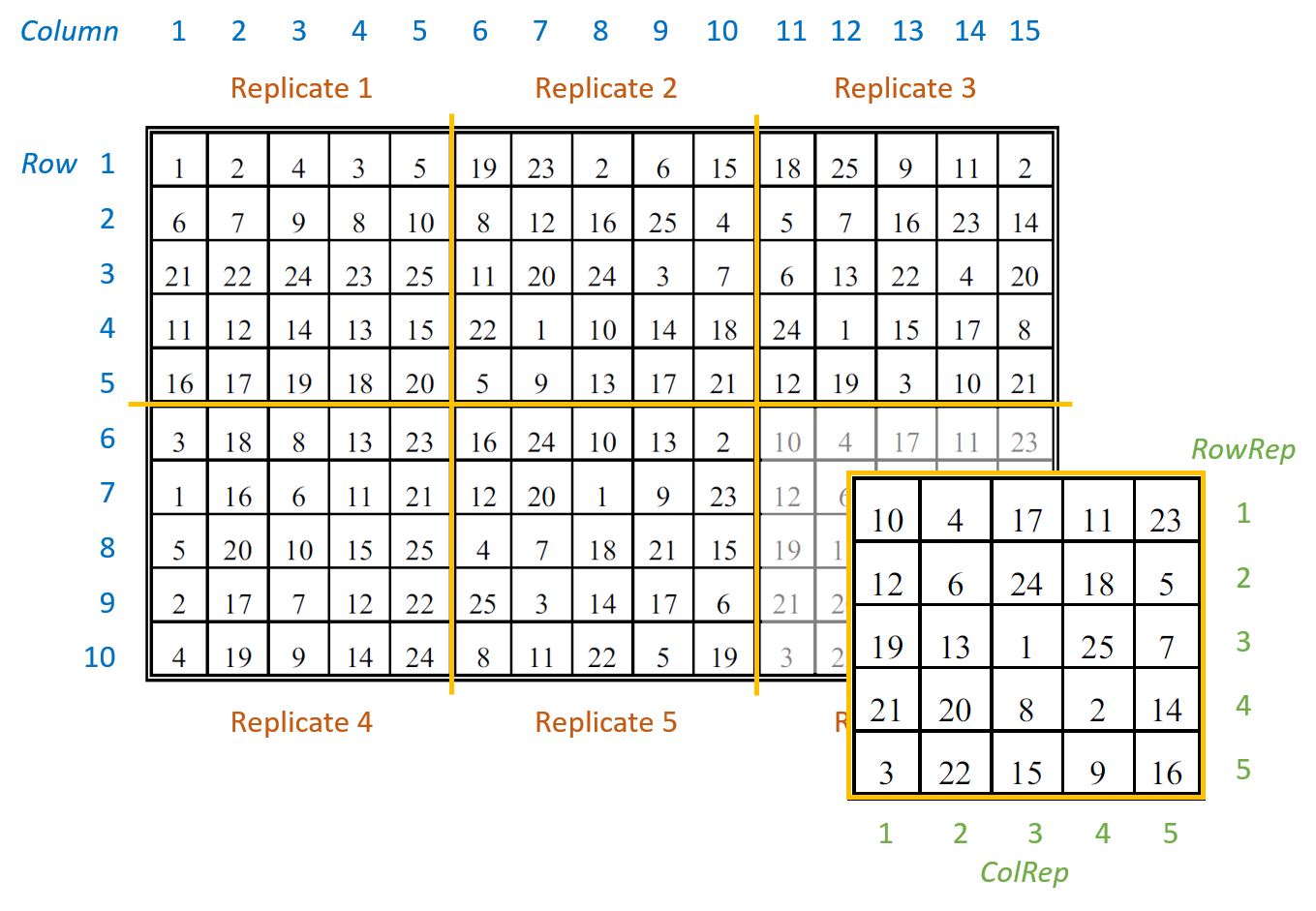There are seven columns in the data frame: five blocking factors (Rep, RowRep, ColRep, Row, Column), one treatment factor, Variety, and the response variate, yield.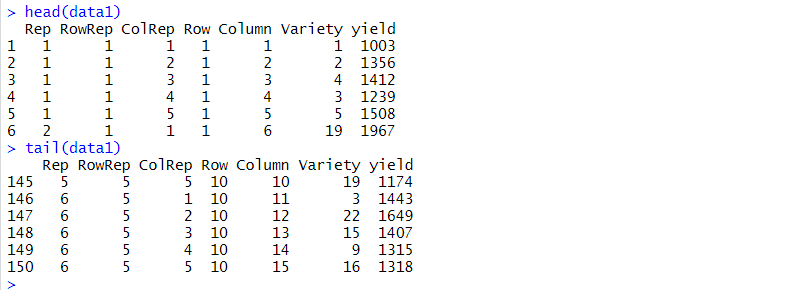The six replicates are numbered from 1 to 6 (Rep). The lattice block numbering is coded within replicates. That is, within each replicates the lattice rows (RowRep) and lattice columns (ColRep) are both numbered from 1 to 5. The Row and Column factors define the row and column positions within the field (rather than within each replicate).

Analysis of a balanced lattice square design

To analyze the response variable, yield, we need to identify the two basic components of the experiment: the treatment structure and the blocking (or design) structure. The treatment structure consists of the set of treatments, or treatment combinations, selected to study or to compare. In our example, there is one treatment factor with 25 levels, Variety (i.e. the 25 different varieties of barley). The blocking structure of replicates (Rep), lattice rows within replicates (Rep:RowRep), and lattice columns within replicates (Rep:ColRep) reflects the balanced lattice square design. In a mixed model analysis, the treatment factors are (usually) fitted as fixed effects and the blocking factors as random.

The balanced lattice square model is fitted in ASReml-R4 using the following code:

> lattice.asr <- asreml(fixed = yield ~ Variety,
random = ~ Rep + Rep:RowRep + Rep:ColRep,
data=data1)

The REML log-likelihood is -707.786.

The model’s BIC is: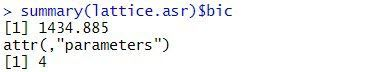The estimated variance components are: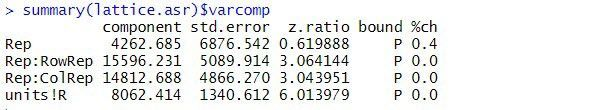The table above contains the estimated variance components for all terms in the random model. The variance component measures the inherent variability of the term, over and above the variability of the sub-units of which it is composed. The variance components for Rep, Rep:RowRep and Rep:ColRep are estimated as 4263, 15596, and 14813, respectively. As is typical, the largest unit (replicate) is more variable than its sub-units (lattice rows and columns within replicates). The "units!R" component is the residual variance.

By default, fixed effects in ASReml-R4 are tested using sequential Wald tests: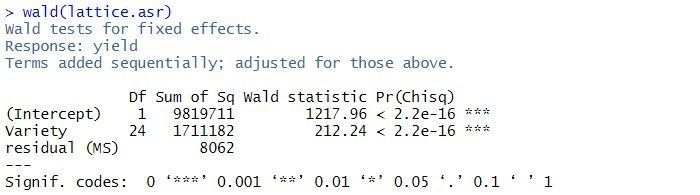In this example, there are two terms in the summary table: the overall mean, (Intercept), and Variety. As the tests are sequential, the effect of the Variety is assessed by calculating the change in sums of squares between the two models (Intercept)+Variety and (Intercept). The p-value (Pr(Chisq)) of  < 2.2 x 10-16 indicates that Variety is a highly significant.

The predicted means for the Variety can be obtained using the predict() function. The standard error of the difference between any pair of variety means is 62. Note: all variety means have the same standard error as the design is balanced.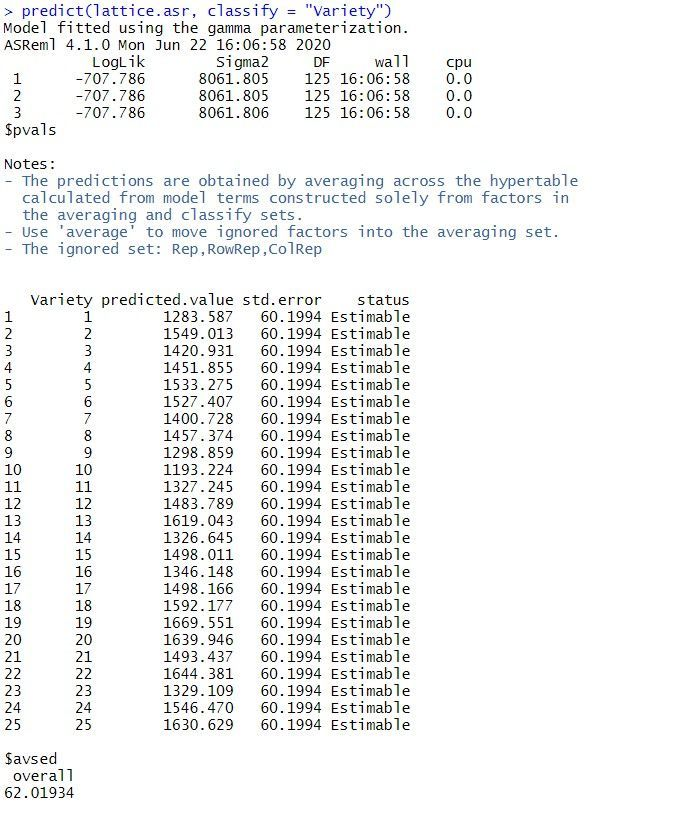Note: the same analysis is obtained when the random model is redefined as replicates (Rep), rows within replicates (Rep:Row) and columns within replicates (Rep:Column).

Spatial analysis of a field experiment

As the plots are laid out in a grid, the data can also be analyzed using a spatial model. We’ll illustrate spatial analysis by fitting a model with a separable first order autoregressive process in the field row (Row) and field column (Column) directions. This is often a useful model to start the spatial modeling process.

The separable first order autoregressive spatial model is fitted in ASReml-R4 using the following code:

> spatial.asr <- asreml(fixed = yield ~ Variety,
residual = ~ar1(Row):ar1(Column),
data = data1)

The BIC for this spatial model is: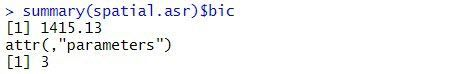The estimated variance components and sequential Wald tests are: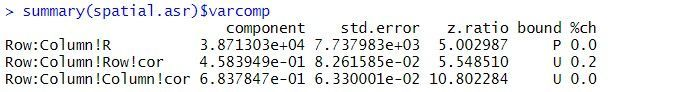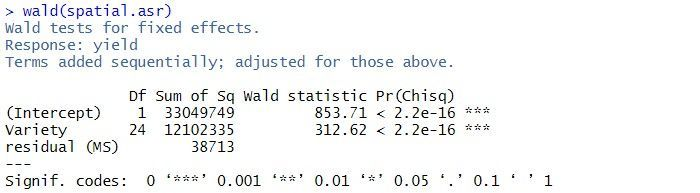The residual variance is 38713, the estimated row correlation is 0.458, and the estimated column correlation is 0.684. As for the balanced lattice square model, there is strong evidence of a Variety effect (p-value < 2.2 x 10-16).

A log-likelihood ratio test cannot be used to compare the balanced lattice square model with the spatial models, as the variance models are not nested. However, the two models can be compared using BIC. As the spatial model has a smaller BIC (1415) than the balanced lattice square model (1435), of the two models explored in this blog, it is chosen as the preferred model. However, selecting the optimal spatial model can be difficult. The current spatial model can be extended by including measurement error (or nugget effect) or revised by selecting a different variance model for the spatial effects.

References

Butler, D.G., Cullis, B.R., Gilmour, A. R., Gogel, B.G. and Thompson, R. (2017). ASReml-R Reference Manual Version 4. VSN International Ltd, Hemel Hempstead, HP2 4TP UK.

Gilmour, A. R., Anderson, R. D. and Rae, A. L. (1995). The analysis of binomial data by a generalised linear mixed model, Biometrika 72: 593-599..The VSNi Team

8 months ago

Should I drop the outliers from my analysis?

Outliers are sample observations that are either much larger or much smaller than the other observations in a dataset. Outliers can skew your dataset, so how should you deal with them?

An example outlier problem

Imagine Jane, the general manager of a chain of computer stores, has asked a statistician, Vanessa, to assist her with the analysis of data on the daily sales at the stores she manages. Vanessa takes a look at the data, and produces a boxplot for each of the stores as shown below.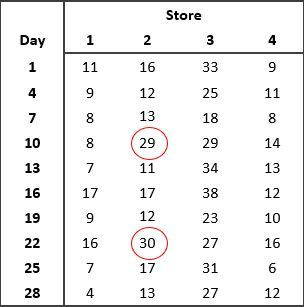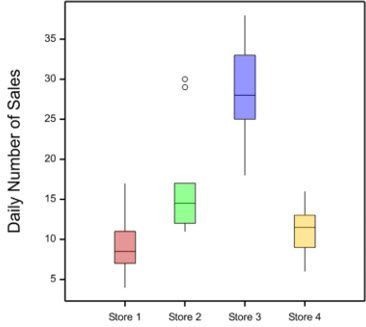What do you notice about the data?

Vanessa pointed out to Jane the presence of outliers in the data from Store 2 on days 10 and 22. Vanessa recommended that Jane checks the accuracy of the data. Are the outliers due to recording or measurement error? If the outliers can’t be attributed to errors in the data, Jane should investigate what might have caused the increased sales on these two particular days. Always investigate outliers - this will help you better understand the data, how it was generated and how to analyse it.

Should we remove the outliers?

Vanessa explained to Jane that we should never drop a data value just because it is an outlier. The nature of the outlier should be investigated before deciding what to do.

Whenever there are outliers in the data, we should look for possible causes of error in the data. If you find an error but cannot recover the correct data value, then you should replace the incorrect data value with a missing value.However, outliers can also be real observations, and sometimes these are the most interesting ones! If your outlier can’t be attributed to an error, you shouldn’t remove it from the dataset. Removing data values unnecessarily, just because they are outliers, introduces bias and may lead you to draw the wrong conclusions from your study.

What should we do if we need/want to keep the outlier?

• Transform the data: if the dataset is not normally distributed, we can try transforming the data to normalize it. For example, if the data set has some high-value outliers (i.e. is right skewed), the log transformation will “pull” the high values in. This often works well for count data.
• Try a different model/analysis: different analyses may make different distributional assumptions, and you should pick one that is appropriate for your data. For example, count data are generally assumed to follow a Poisson distribution. Alternatively, the outliers may be able to be modelled using an appropriate explanatory variable. For example, computer sales may increase as we approach the start of a new school year.

In our example, Vanessa suggested that since the mean for Store 2 is highly influenced by the outliers, the median, another measure of central tendency, seems more appropriate for summarizing the daily sales at each store. Using the statistical software Genstat, Vanessa can easily calculate both the mean and median number of sales per store for Jane.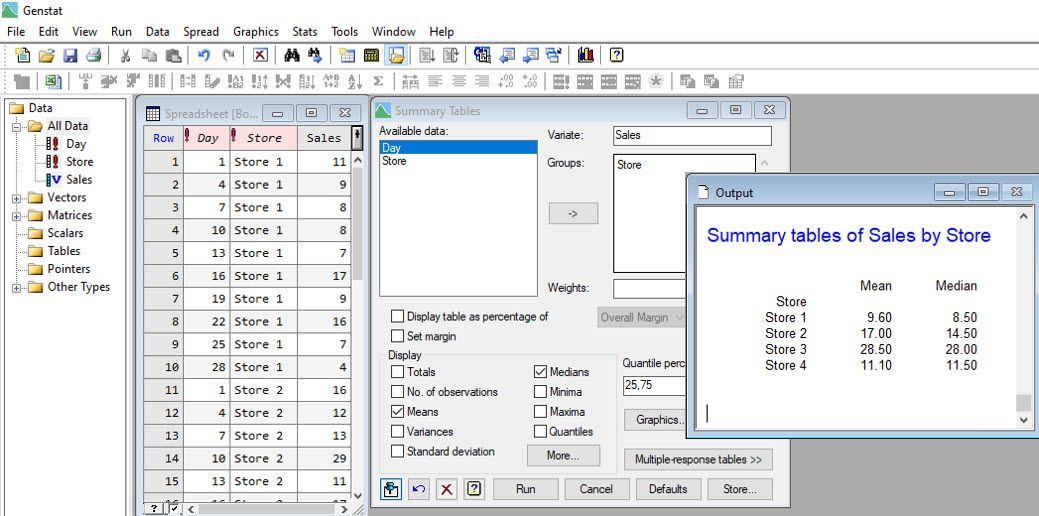Vanessa also analyses the data assuming the daily sales have Poisson distributions, by fitting a log-linear model.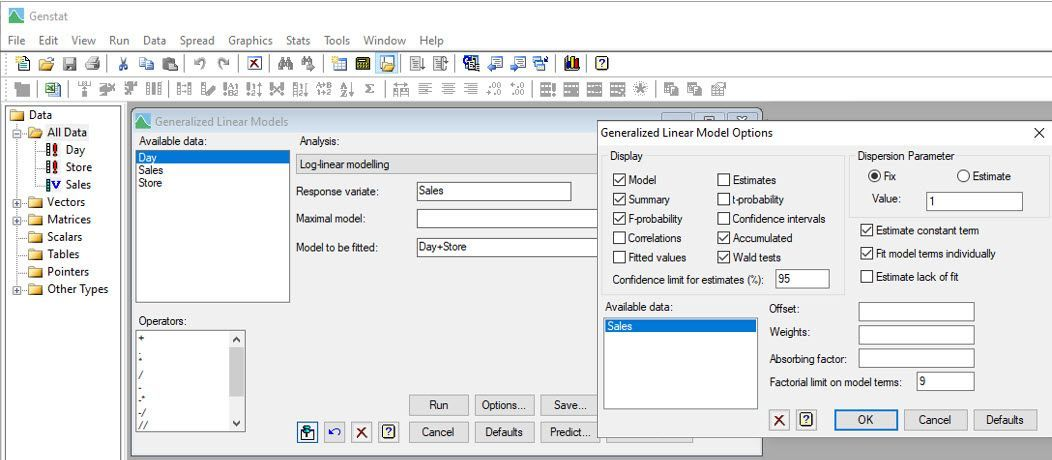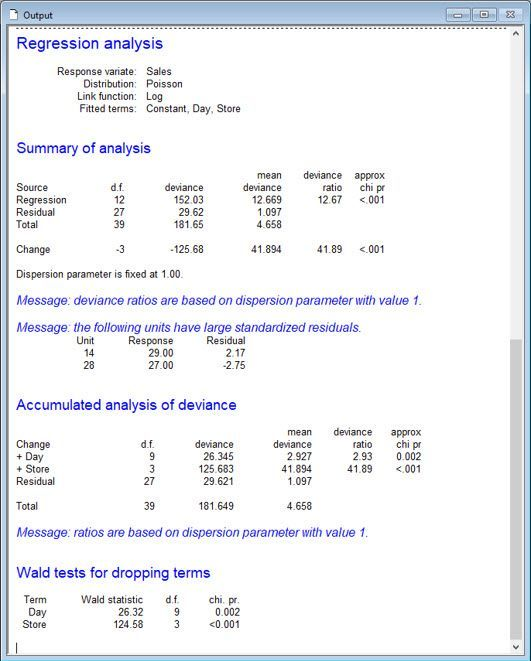Notice that Vanessa has included “Day” as a blocking factor in the model to allow for variability due to temporal effects.

From this analysis, Vanessa and Jane conclude that the means (of the Poisson distributions) differ between the stores (p-value < 0.001). Store 3, on average, has the most computer sales per day, whereas Stores 1 and 4, on average, have the least.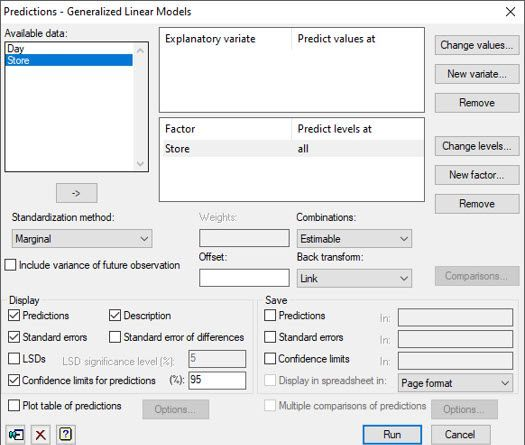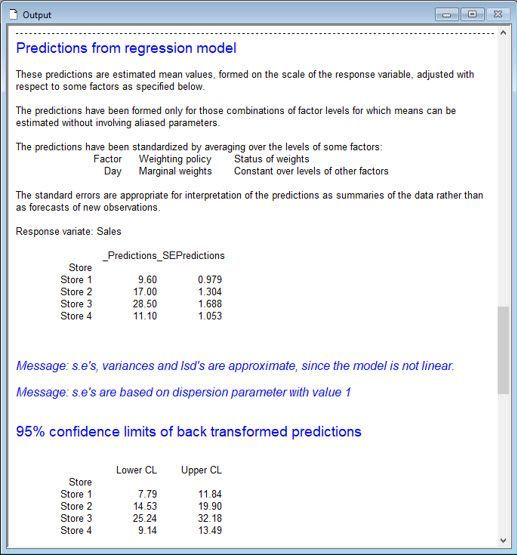There are other statistical approaches Vanessa might have used to analyse Jane’s sales data, including a one-way ANOVA blocked by Day on the log-transformed sales data and Friedman’s non-parametric ANOVA. Both approaches are available in Genstat’s comprehensive menu system.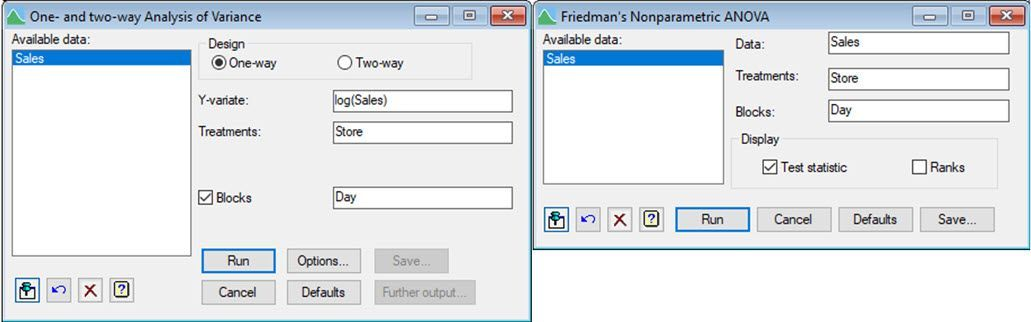What is the best method to deal with outliers?

There are many ways to deal with outliers, but no single method will work in every situation. As we have learnt, we can remove an observation if we have evidence it is an error. But, if that is not the case, we can always use alternative summary statistics, or even different statistical approaches, that accommodate them.